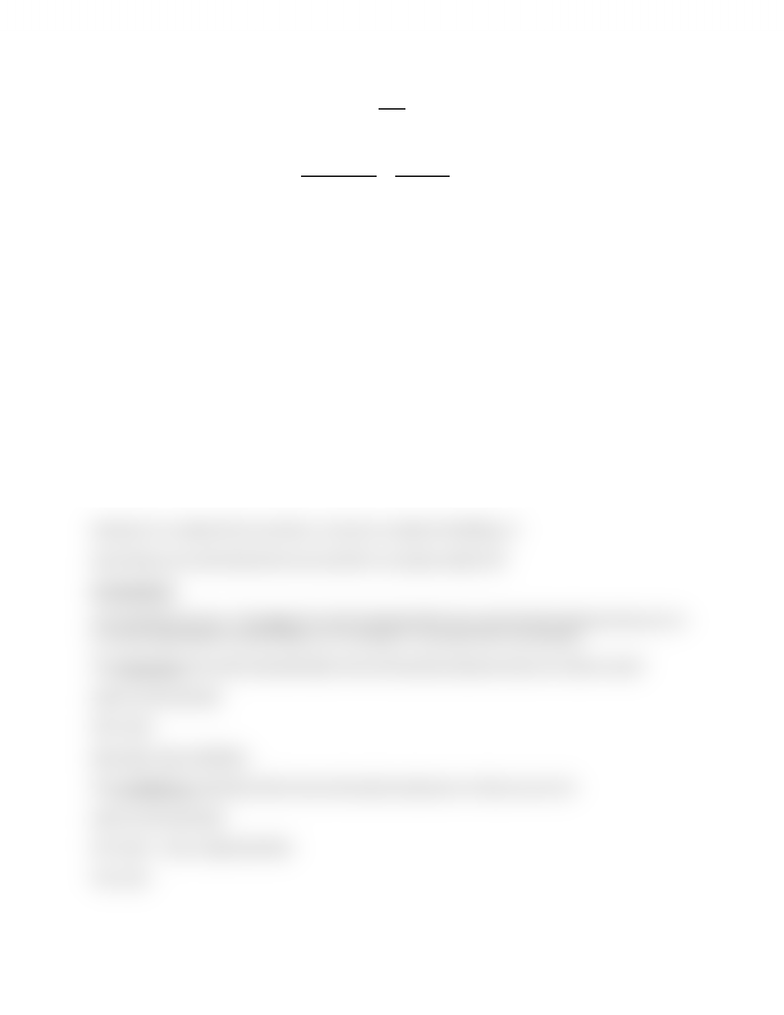# MA100 Lecture Notes - Subset, Natural Number, Empty Set

44 views2 pages
School
WLU
Department
Mathematics
Course
MA100
ProfessorSets
A set is a collection of objects called the elements or members of the set.
Ex. A= {Alice, bob, Cameron} - order doesn’t matter
B= {2,3,4,5,6} - no repetition
Sets can be written using a property B= {integers X such that 2≤X≤6}
= {integers X: 2≤X≤6}
2εB – 2 is in the set
10ε\B 10 is not in the set
A set that has no elements is called the empty set or the void set and is denoted φ
Two sets are equal if they have exactly the same elements
If C= {6, 3, 4, 5, 2} then C=B
If D= {2, 3, 4, 5, 7} then D≠B
If E= {2, 3, 4, 5} then E≠B
However E is a subset of B, we write Eʗ_ B (a set is a subset of itself) φʗ_ B
Since E≠B we can write EʗB and we can say that E is a proper subset of B
Set operations
Let A and B be two sets. The union of A and B, denoted AUB is the set formed by elements that are in A
or in B (or both) AUB= {X: XεA or XεB} ex. X= {2,4,6,8} Y= {2,3,5,8} XUY= {2,3,4,5,6,8}
The intersection of A and B, denoted AηB is the set formed by elements that are in both A and B
AηB= {X: XεA and XεB}
XηY= {2,8}
Note AηB = BηA, AUB=BUA
The set-difference, denoted, A\B is the set formed by elements in A that are not in B
A\B= {X: XεA and Xε/B}
X\Y= {4,6} - note: usually A\B ≠B\A
Y\X= {3,5}
Unlock document

This preview shows half of the first page of the document.
Unlock all 2 pages and 3 million more documents.